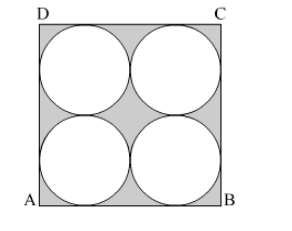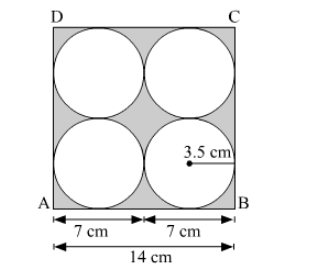# In the given figure, find the area of the shaded region, where ABCD is a square of side 14 cm`
Question:

In the given figure, find the area of the shaded region, where ABCD is a square of side 14 cm and all circles are of the same diameter.Solution:Area of the square $=\operatorname{Side}^{2}=14^{2}=196$ sq. $\mathrm{cm}$

Area of the circles $=4 \times \pi \times 3.5 \times 3.5=154$ sq. $\mathrm{cm}$

Area of the shaded region = Area of the square -">Area of four circles

$=196-154$

$=42 \mathrm{~cm}^{2}$